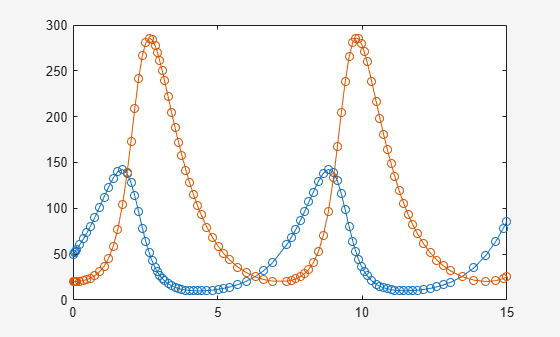Main Content

# massMatrixForm

Extract mass matrix and right side of semilinear system of differential algebraic equations

## Syntax

``````[M,F] = massMatrixForm(eqs,vars)``````

## Description

example

``````[M,F] = massMatrixForm(eqs,vars)``` returns the mass matrix `M` and the right side of equations `F` of a semilinear system of first-order differential algebraic equations (DAEs). Algebraic equations in `eqs` that do not contain any derivatives of the variables in `vars` correspond to empty rows of the mass matrix `M`.The mass matrix `M` and the right side of equations `F` refer to this form.$M\left(t,x\left(t\right)\right)\stackrel{˙}{x}\left(t\right)=F\left(t,x\left(t\right)\right).$```

## Examples

collapse all

Convert a semilinear system of differential algebraic equations to mass matrix form.

Create the following system of differential algebraic equations. Here, the functions `x1(t)` and `x2(t)` represent state variables of the system. The system also contains symbolic parameters `r` and `m`, and the function `f(t,x1,x2)`. Specify the equations and variables as two symbolic vectors: equations as a vector of symbolic equations, and variables as a vector of symbolic function calls.

```syms x1(t) x2(t) f(t,x1,x2) r m eqs = [m*x2(t)*diff(x1(t), t) + m*t*diff(x2(t), t) == f(t, x1(t), x2(t)),... x1(t)^2 + x2(t)^2 == r^2]; vars = [x1(t),x2(t)];```

Find the mass matrix form of this system.

`[M,F] = massMatrixForm(eqs, vars)`
```M =  $\left(\begin{array}{cc}m {x}_{2}\left(t\right)& m t\\ 0& 0\end{array}\right)$```
```F =  $\left(\begin{array}{c}f\left(t,{x}_{1}\left(t\right),{x}_{2}\left(t\right)\right)\\ {r}^{2}-{{x}_{1}\left(t\right)}^{2}-{{x}_{2}\left(t\right)}^{2}\end{array}\right)$```

Solve this system using the numerical solver `ode15s`. Before you use `ode15s`, assign the following values to symbolic parameters of the system: `m = 100`, `r = 1`, `f(t,x1,x2) = t + x1*x2`. Also, replace the state variables `x1(t)`, `x2(t)` by variables `Y1`, `Y2` acceptable by `matlabFunction`.

```syms Y1 Y2 M = subs(M, [vars, m, r, f], [Y1, Y2, 100, 1, @(t,x1,x2) t + x1*x2]); F = subs(F, [vars, m, r, f], [Y1, Y2, 100, 1, @(t,x1,x2) t + x1*x2]);```

Create the following function handles `MM` and `FF`. You can use these function handles as input arguments for `odeset` and `ode15s`. These functions require state variables to be specified as column vectors.

```MM = matlabFunction(M, 'vars', {t, [Y1; Y2]}); FF = matlabFunction(F, 'vars', {t, [Y1; Y2]});```

Solve the system using `ode15s`.

```opt = odeset('Mass', MM, 'InitialSlope', [0.005;0]); ode15s(FF, [0,1], [0.5; 0.5*sqrt(3)], opt)```Copyright 2014 The MathWorks, Inc

## Input Arguments

collapse all

System of semilinear first-order DAEs, specified as a vector of symbolic equations or expressions.

State variables, specified as a vector of symbolic functions or function calls, such as `x(t)`.

Example: `[x(t),y(t)]` or `[x(t);y(t)]`

## Output Arguments

collapse all

Mass matrix of the system, returned as a symbolic matrix. The number of rows is the number of equations in `eqs`, and the number of columns is the number of variables in `vars`.

Right sides of equations, returned as a column vector of symbolic expressions. The number of elements in this vector is equal to the number of equations in `eqs`.

## See Also

### Topics

Introduced in R2014b

## Support

#### Mathematical Modeling with Symbolic Math Toolbox

Get examples and videos